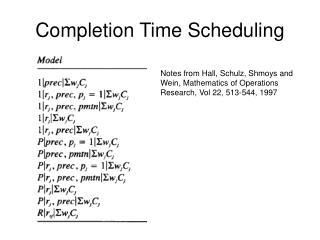DownloadDownload PresentationCompletion Time Scheduling

# Completion Time Scheduling

Download Presentation## Completion Time Scheduling

- - - - - - - - - - - - - - - - - - - - - - - - - - - E N D - - - - - - - - - - - - - - - - - - - - - - - - - - -
##### Presentation Transcript

1. Completion Time Scheduling Notes from Hall, Schulz, Shmoys and Wein, Mathematics of Operations Research, Vol 22, 513-544, 1997

2. One LP formulation for 1||ΣwjCj

3. Other ways to bound Cj Smith’s rule: Scheduling jobs by wj/pj is guaranteed to be optimal wj pj

4. Think of all jobs as having wj = pj Smith’s rule: Any order is then equivalent since wj/pj = 1 for all jobs pj pj

5. 1|prec| ΣwjCj • LP: minimize ΣwjCj • Subject to Ck ≥ Cj + pk (if job j precedes job k) Let C’i denote the LP optimal values Let C*i denote the true optimal values

6. Algorithm and Analysis • Let C’i denote the LP optimal values • Let C*i denote the true optimal values • Greedy Algorithm: • Solve LP for C’i • Solvable in poly time despite exponential size • Prioritize the jobs by C’i values • Let Gi be the resulting completion times • Key result: Gi ≤ 2C’i ≤ 2C*i • From Lemma 2.1

7. 1|rj, prec| ΣwjCj • LP: minimize ΣwjCj • Subject to Cj ≥ rj + pj (all jobs) Ck ≥ Cj + pk (if job j precedes job k) Let C’i denote the LP optimal values Let C*i denote the true optimal values

8. Algorithm • Let C’i denote the LP optimal values • Let C*i denote the true optimal values • Greedy Algorithm: • Solve LP for C’i • Solvable in poly time despite exponential size • Prioritize the jobs by C’i values • Let Gi be the resulting completion times

9. Analysis • Key result: Gi ≤ 3C’i ≤ 3C*i • Fix j and define S = {1, …, j} • Gj ≤ rmax(S) + p(S) • C’j ≥ rmax(S) • Thus Gj ≤ C’j + p(S) ≤ 3C’j • From Lemma 2.1

10. Extending to parallel machines

11. Extending to parallel machines

12. P|rj| ΣwjCj • LP: minimize ΣwjCj • Subject to Cj ≥ rj + pj Let C’i denote the LP optimal values Let C*i denote the true optimal values

13. Algorithm • Let C’i denote the LP optimal values • Let C*i denote the true optimal values • Greedy Algorithm: • Solve LP for C’i • Solvable in poly time despite exponential size • Prioritize the jobs by C’i values • Let Gi be the resulting completion times

14. Analysis • Key result: Gi ≤ (4-1/m)C’i ≤ 4C*i • Fix j and define S = {1, …, j} • Gj ≤ rmax(S) + 1/m p(S – {j}) + pj • = rmax(S) + 1/m p(S) + (1-1/m)pj

15. Preemption vs Nonpreemption • Method for converting preemptive schedules into non-preemptive schedules • Effective for minimizing Cj objectives • Prioritize jobs by their preemptive completion times Cjp • Generalization: When α of the job is complete • List schedule these jobs nonpreemptively using this priority

16. 1 machine conversion • Let Cpi denote any preemptive values • Let Cni denote the nonpreemptive values • Cni ≤ 2 Cpi CPj …

17. 1|rj| ΣCj • With preemption, we have an optimal solution, SRPT • Nonpreemptive online: • Simulate SRPT and when a job is completed in SRPT, start it in the non-preemptive (or add it to the list to start)